# Find the Side of the smallest Square that can contain given 4 Big Squares

Given sides of four small squares. You have to find the side of the smallest square such that it can contain all given 4 squares without overlapping. The side of a square can be up to 10^16.

Examples:

```Input: side1 = 2, side2 = 2, side3 = 2, side4 = 2
Output: 4

Input: side1 = 100000000000000, side2 = 123450000000000,
side3 = 987650000000000, side4 = 987654321000000
Output: 1975304321000000
```

## Recommended: Please try your approach on {IDE} first, before moving on to the solution.

Approach:
It is given that no two squares will overlap. Therefore to find the side of the smallest suitable square, we will find all four sides, when squares will be put in 2 x 2 manner. That is 2 squares will be side by side and rest 2 will be put together.

So we calculate all four side and select one that will be maximum.

Example: When all small squares are of same side.

1.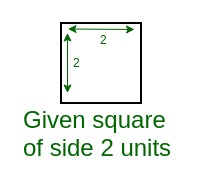2.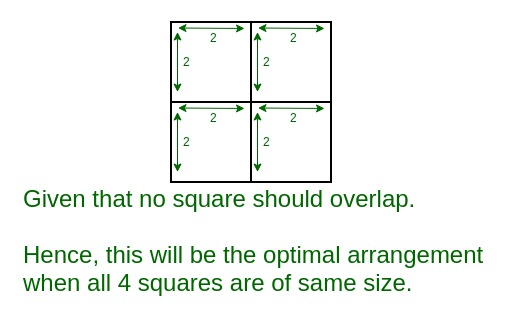3.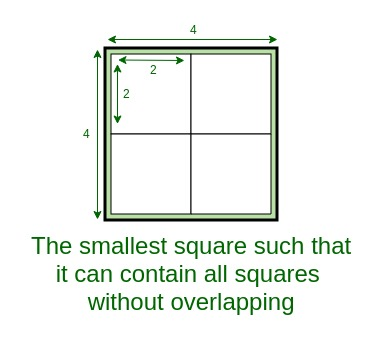Example: When all small squares are of different side.

1.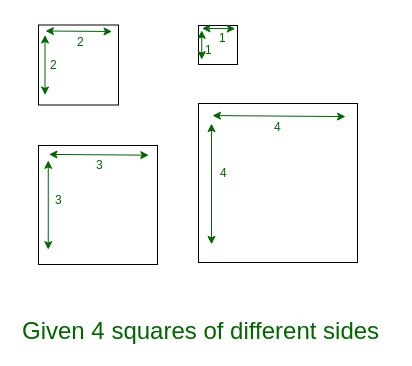2.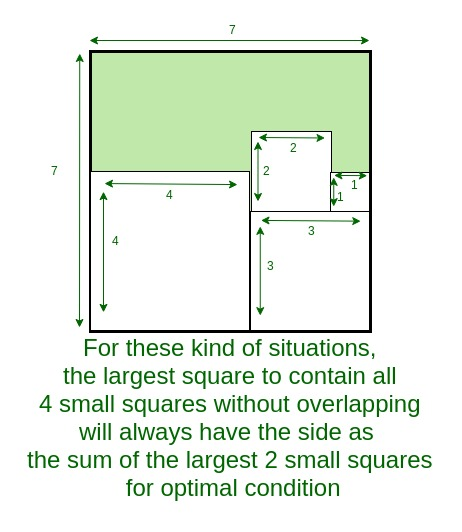Below is the implementation of the above approach:

## C++

 `// C++ program to Find the Side ` `// of the smallest Square ` `// that can contain given 4 Big Squares ` ` `  `#include ` `using` `namespace` `std; ` ` `  `// Function to find the maximum of two values ` `long` `long` `int` `max(``long` `long` `a, ``long` `long` `b) ` `{ ` `    ``if` `(a > b) ` `        ``return` `a; ` `    ``else` `        ``return` `b; ` `} ` ` `  `// Function to find the smallest side ` `// of the suitable suitcase ` `long` `long` `int` `smallestSide(``long` `long` `int` `a[]) ` `{ ` `    ``// sort array to find the smallest ` `    ``// and largest side of suitcases ` `    ``sort(a, a + 4); ` ` `  `    ``long` `long` `side1, side2, side3, side4, ` `        ``side11, side12, sideOfSquare; ` ` `  `    ``// side of the suitcase will be smallest ` `    ``// if they arranged in 2 x 2 way ` `    ``// so find all possible sides of that arrangement ` `    ``side1 = a + a; ` `    ``side2 = a + a; ` `    ``side3 = a + a; ` `    ``side4 = a + a; ` ` `  `    ``// since suitcase should be square ` `    ``// so find maximum of all four side ` `    ``side11 = max(side1, side2); ` `    ``side12 = max(side3, side4); ` ` `  `    ``// now find greatest side and ` `    ``// that will be the smallest square ` `    ``sideOfSquare = max(side11, side12); ` ` `  `    ``// return the result ` `    ``return` `sideOfSquare; ` `} ` ` `  `// Driver program ` `int` `main() ` `{ ` `    ``long` `long` `int` `side; ` ` `  `    ``cout << ``"Test Case 1\n"``; ` ` `  `    ``// Get the side of the 4 small squares ` `    ``side = 2; ` `    ``side = 2; ` `    ``side = 2; ` `    ``side = 2; ` ` `  `    ``// Find the smallest side ` `    ``cout << smallestSide(side) << endl; ` ` `  `    ``cout << ``"\nTest Case 2\n"``; ` ` `  `    ``// Get the side of the 4 small squares ` `    ``side = 100000000000000; ` `    ``side = 123450000000000; ` `    ``side = 987650000000000; ` `    ``side = 987654321000000; ` ` `  `    ``// Find the smallest side ` `    ``cout << smallestSide(side) << endl; ` ` `  `    ``return` `0; ` `} `

## Java

 `// Java program to Find the Side ` `// of the smallest Square that  ` `// can contain given 4 Big Squares ` `// Java implementation of the approach ` `import` `java.util.Arrays; ` ` `  `class` `GFG  ` `{ ` ` `  `// Function to find the maximum of two values ` `static` `long` `max(``long` `a, ``long` `b) ` `{ ` `    ``if` `(a > b) ` `        ``return` `a; ` `    ``else` `        ``return` `b; ` `} ` ` `  `// Function to find the smallest side ` `// of the suitable suitcase ` `static` `long` `smallestSide(``long` `a[]) ` `{ ` `    ``// sort array to find the smallest ` `    ``// and largest side of suitcases ` `    ``Arrays.sort(a); ` ` `  `    ``long` `side1, side2, side3, side4, ` `        ``side11, side12, sideOfSquare; ` ` `  `    ``// side of the suitcase will be smallest ` `    ``// if they arranged in 2 x 2 way ` `    ``// so find all possible sides of that arrangement ` `    ``side1 = a[``0``] + a[``3``]; ` `    ``side2 = a[``1``] + a[``2``]; ` `    ``side3 = a[``0``] + a[``1``]; ` `    ``side4 = a[``2``] + a[``3``]; ` ` `  `    ``// since suitcase should be square ` `    ``// so find maximum of all four side ` `    ``side11 = max(side1, side2); ` `    ``side12 = max(side3, side4); ` ` `  `    ``// now find greatest side and ` `    ``// that will be the smallest square ` `    ``sideOfSquare = max(side11, side12); ` ` `  `    ``// return the result ` `    ``return` `sideOfSquare; ` `} ` ` `  `// Driver program ` `public` `static` `void` `main(String[] args)  ` `{ ` `    ``long` `side[] = ``new` `long``[``4``]; ` ` `  `    ``System.out.println(``"Test Case 1"``); ` ` `  `    ``// Get the side of the 4 small squares ` `    ``side[``0``] = ``2``; ` `    ``side[``1``] = ``2``; ` `    ``side[``2``] = ``2``; ` `    ``side[``3``] = ``2``; ` ` `  `    ``// Find the smallest side ` `    ``System.out.println(smallestSide(side)); ` ` `  `    ``System.out.println(``"\nTest Case 2"``); ` ` `  `    ``// Get the side of the 4 small squares ` `    ``side[``0``] = 100000000000000L; ` `    ``side[``1``] = 123450000000000L; ` `    ``side[``2``] = 987650000000000L; ` `    ``side[``3``] = 987654321000000L; ` ` `  `    ``// Find the smallest side ` `    ``System.out.println(smallestSide(side)); ` ` `  `    ``} ` `} ` ` `  `/* This code contributed by PrinciRaj1992 */`

## Python3

 `# Python 3 program to Find the Side ` `# of the smallest Square that   ` `# can contain given 4 Big Squares ` ` `  `# Function to find the maximum  ` `# of two values ` `def` `max``(a, b): ` `    ``if` `(a > b): ` `        ``return` `a ` `    ``else``: ` `        ``return` `b ` ` `  `# Function to find the smallest side ` `# of the suitable suitcase ` `def` `smallestSide(a): ` `     `  `    ``# sort array to find the smallest ` `    ``# and largest side of suitcases ` `    ``a.sort(reverse ``=` `False``) ` ` `  `    ``# side of the suitcase will be  ` `    ``# smallest if they arranged in  ` `    ``# 2 x 2 way so find all possible ` `    ``# sides of that arrangement ` `    ``side1 ``=` `a[``0``] ``+` `a[``3``] ` `    ``side2 ``=` `a[``1``] ``+` `a[``2``] ` `    ``side3 ``=` `a[``0``] ``+` `a[``1``] ` `    ``side4 ``=` `a[``2``] ``+` `a[``3``] ` ` `  `    ``# since suitcase should be square ` `    ``# so find maximum of all four side ` `    ``side11 ``=` `max``(side1, side2) ` `    ``side12 ``=` `max``(side3, side4) ` ` `  `    ``# now find greatest side and ` `    ``# that will be the smallest square ` `    ``sideOfSquare ``=` `max``(side11, side12) ` ` `  `    ``# return the result ` `    ``return` `sideOfSquare ` ` `  `# Driver Code ` `if` `__name__ ``=``=` `'__main__'``: ` `    ``side ``=` `[``0` `for` `i ``in` `range``(``4``)] ` ` `  `    ``print``(``"Test Case 1"``) ` ` `  `    ``# Get the side of the 4 ` `    ``# small squares ` `    ``side[``0``] ``=` `2` `    ``side[``1``] ``=` `2` `    ``side[``2``] ``=` `2` `    ``side[``3``] ``=` `2` ` `  `    ``# Find the smallest side ` `    ``print``(smallestSide(side)) ` ` `  `    ``print``(``"\n"``, end ``=` `"") ` `    ``print``(``"Test Case 2"``) ` ` `  `    ``# Get the side of the 4 small squares ` `    ``side[``0``] ``=` `100000000000000` `    ``side[``1``] ``=` `123450000000000` `    ``side[``2``] ``=` `987650000000000` `    ``side[``3``] ``=` `987654321000000` ` `  `    ``# Find the smallest side ` `    ``print``(smallestSide(side)) ` ` `  `# This code is contributed by ` `# Surendra_Gangwar `

## C#

 `// C# program to Find the Side ` `// of the smallest Square that  ` `// can contain given 4 Big Squares ` `// Java implementation of the approach ` `using` `System; ` `     `  `class` `GFG  ` `{ ` ` `  `// Function to find the maximum of two values ` `static` `long` `max(``long` `a, ``long` `b) ` `{ ` `    ``if` `(a > b) ` `        ``return` `a; ` `    ``else` `        ``return` `b; ` `} ` ` `  `// Function to find the smallest side ` `// of the suitable suitcase ` `static` `long` `smallestSide(``long` `[]a) ` `{ ` `    ``// sort array to find the smallest ` `    ``// and largest side of suitcases ` `    ``Array.Sort(a); ` ` `  `    ``long` `side1, side2, side3, side4, ` `        ``side11, side12, sideOfSquare; ` ` `  `    ``// side of the suitcase will be smallest ` `    ``// if they arranged in 2 x 2 way ` `    ``// so find all possible sides of that arrangement ` `    ``side1 = a + a; ` `    ``side2 = a + a; ` `    ``side3 = a + a; ` `    ``side4 = a + a; ` ` `  `    ``// since suitcase should be square ` `    ``// so find maximum of all four side ` `    ``side11 = max(side1, side2); ` `    ``side12 = max(side3, side4); ` ` `  `    ``// now find greatest side and ` `    ``// that will be the smallest square ` `    ``sideOfSquare = max(side11, side12); ` ` `  `    ``// return the result ` `    ``return` `sideOfSquare; ` `} ` ` `  `// Driver code ` `public` `static` `void` `Main(String[] args)  ` `{ ` `    ``long` `[]side = ``new` `long``; ` ` `  `    ``Console.WriteLine(``"Test Case 1"``); ` ` `  `    ``// Get the side of the 4 small squares ` `    ``side = 2; ` `    ``side = 2; ` `    ``side = 2; ` `    ``side = 2; ` ` `  `    ``// Find the smallest side ` `    ``Console.WriteLine(smallestSide(side)); ` ` `  `    ``Console.WriteLine(``"\nTest Case 2"``); ` ` `  `    ``// Get the side of the 4 small squares ` `    ``side = 100000000000000L; ` `    ``side = 123450000000000L; ` `    ``side = 987650000000000L; ` `    ``side = 987654321000000L; ` ` `  `    ``// Find the smallest side ` `    ``Console.WriteLine(smallestSide(side)); ` `} ` `} ` ` `  `// This code contributed by Rajput-Ji `

## PHP

 ` ``\$b``) ` `        ``return` `\$a``; ` `    ``else` `        ``return` `\$b``; ` `} ` ` `  `// Function to find the smallest side ` `// of the suitable suitcase ` `function` `smallestSide(``\$a``) ` `{ ` `    ``// sort array to find the smallest ` `    ``// and largest side of suitcases ` `    ``sort(``\$a``, 0); ` ` `  `    ``// side of the suitcase will be smallest ` `    ``// if they arranged in 2 x 2 way ` `    ``// so find all possible sides of that arrangement ` `    ``\$side1` `= ``\$a`` + ``\$a``; ` `    ``\$side2` `= ``\$a`` + ``\$a``; ` `    ``\$side3` `= ``\$a`` + ``\$a``; ` `    ``\$side4` `= ``\$a`` + ``\$a``; ` ` `  `    ``// since suitcase should be square ` `    ``// so find maximum of all four side ` `    ``\$side11` `= max1(``\$side1``, ``\$side2``); ` `    ``\$side12` `= max1(``\$side3``, ``\$side4``); ` ` `  `    ``// now find greatest side and ` `    ``// that will be the smallest square ` `    ``\$sideOfSquare` `= max1(``\$side11``, ``\$side12``); ` ` `  `    ``// return the result ` `    ``return` `\$sideOfSquare``; ` `} ` ` `  `// Driver program ` `\$side` `= ``array``(); ` ` `  `echo` `"Test Case 1\n"``; ` ` `  `// Get the side of the 4 small squares ` `\$side`` = 2; ` `\$side`` = 2; ` `\$side`` = 2; ` `\$side`` = 2; ` ` `  `// Find the smallest side ` `echo` `smallestSide(``\$side``) . ``"\n"``; ` ` `  `echo` `"\nTest Case 2\n"``; ` ` `  `// Get the side of the 4 small squares ` `\$side`` = 100000000000000; ` `\$side`` = 123450000000000; ` `\$side`` = 987650000000000; ` `\$side`` = 987654321000000; ` ` `  `// Find the smallest side ` `echo` `smallestSide(``\$side``) . ``"\n"``; ` ` `  `// This code is contributed ` `// by Akanksha Rai ` `?> `

Output:

```Test Case 1
4

Test Case 2
1975304321000000
```

My Personal Notes arrow_drop_upCheck out this Author's contributed articles.

If you like GeeksforGeeks and would like to contribute, you can also write an article using contribute.geeksforgeeks.org or mail your article to contribute@geeksforgeeks.org. See your article appearing on the GeeksforGeeks main page and help other Geeks.

Please Improve this article if you find anything incorrect by clicking on the "Improve Article" button below.